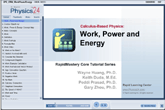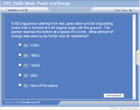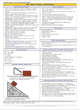How to Learn in 24 Hours?The Rapid Learning Movie

 Need Help? M-F: 9am-5pm(PST): Toll-Free: (877) RAPID-10 US Direct: (714) 692-2900 Int'l: 001-714-692-2900 24/7 Online Technical Support: The Rapid Support Center Secure Online Order:Got Questions? Frequently Asked Questions
 Need Proof? Testimonials by Our Users
 Trustlink is a Better Business Bureau Program. Rapid Learning Center is a fivr-star business. External TrustLink Reviews

 Rapid Learning Courses: MCAT in 24 Hours (2015-16) USMLE in 24 Hours (Boards) Chemistry in 24 Hours Biology in 24 Hours Physics in 24 Hours Mathematics in 24 Hours Psychology in 24 Hours SAT in 24 Hours ACT in 24 Hours AP in 24 Hours CLEP in 24 Hours DAT in 24 Hours (Dental) OAT in 24 Hours (Optometry) PCAT in 24 Hours (Pharmacy) Nursing Entrance Exams Certification in 24 Hours eBook - Survival Kits Audiobooks (MP3)

 Tell-A-Friend: Have friends taking science and math courses too? Tell them about our rapid learning system.Home »  Physics »  Calculus Physics

Work, Power and Energy

 Topic Review on "Title": Work: The work, W, done by a constant force on an object is defined as the product of the component of the force along the direction of displacement and the magnitude of the displacement. Calculation of Work: When calculating work, only the force that is applied in the direction of motion is considered.  W=Fdcos? Postive Work: If the force and displacement are in the same direction, that would be considered positive work. Power: Power is a measure of how quickly work is done. Kinetic Energy: Kinetic energy is energy of motion.  All moving object possess kinetic energy. Gravitational Potential Energy: Gravitational Potential energy is the energy an object possessed due to its position. Base Level: The point that height is measured from.  Any point can be used as a base level because the energy amount you calculate will be relative. Conservation of Energy: Energy cannot be created or destroyed; it may be transformed from one form into another, but the total amount of energy never changes. Conservative Force: A force is classified as conservative if the work it does on a moving object is independent of the path between the initial and final position, OR it does no net work on an object moving around a closed path, starting and finishing at the same point.  Friction or air resistance is an example of a non conservative force.

Rapid Study Kit for "Title":
 Flash Movie Flash Game Flash Card Core Concept Tutorial Problem Solving Drill Review Cheat Sheet"Title" Tutorial Summary : This tutorial will show you how to calculate work.  Additionally, if the time taken to complete this work is known, the power generated can be found too.  Work is related to energy.  In fact, work is defined as the change in energy.  This is the work energy theorem.  This energy can come in many forms. Two of the main types of mechanical energy are kinetic and potential.  Kinetic energy is energy of motion, while potential energy is stored energy.  In many situations, conservation of energy can be applied to help solve problems.  This says that energy isn’t created or destroyed, just transferred from one type to another.

 Tutorial Features: Specific Tutorial Features: Problem-solving techniques are used to work out and illustrate the example problems, step by step. Easy explanation for sometimes confusing physics formulas. Animation showing conservation of energy by a skydiver. Series Features: Concept map showing inter-connections of new concepts in this tutorial and those previously introduced. Definition slides introduce terms as they are needed. Visual representation of concepts Animated examples—worked out step by step A concise summary is given at the conclusion of the tutorial.

 "Title" Topic List: Work Direction of force Sign conventions Power Calculation of power Energy Kinetic energy Potential energy Work energy theorem Conservation of energy Conservative and non conservative forces

See all 24 lessons in high school  physics, including concept tutorials, problem drills and cheat sheets:
Teach Yourself Calculus Physics Visually in 24 Hours